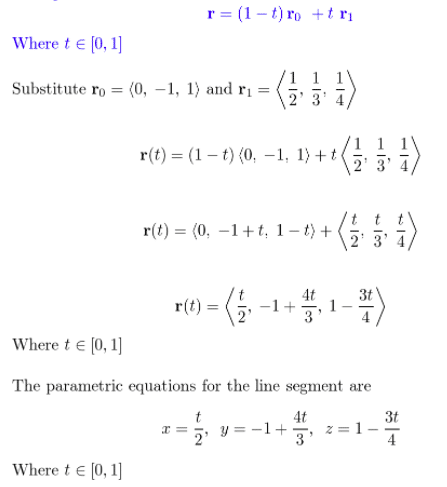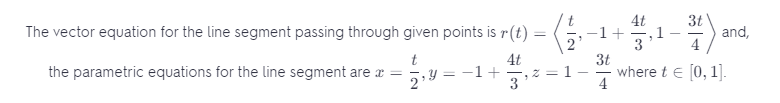# Find A Vector Equation And Parametric Equations For The Line Segment That Joins P To Q.

We thoroughly check each answer to a question to provide you with the most correct answers. Found a mistake? Let us know about it through the REPORT button at the bottom of the page.

Find a vector equation and parametric equations for the line segment that joins P to Q.
P(0, – 1, 1), Q(1/2, 1/3, 1/4)